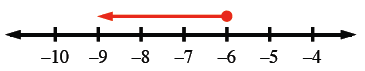Home > CC2MN > Chapter cc18 > Lesson cc18.1.3 > Problem8-43

8-43.

Solve and graph the following inequalities: Homework Help ✎

1. $x - 9 < 17$

• Solve the inequalities like an equation. Substitute the inequality symbol with an equal sign.
$x − 9 = 17$

• Isolate the x by adding nine to each side.
$x − 9 + 9 = 17 + 9$
$x = 26$

• Choose two numbers, one less than $26$ and one greater than $26$. (Example: 3 and 45) Substitute these numbers for x to decide which direction the arrow should point.
$x < 26$
$45 < 26$ (False)
$3 < 26$ (True)
Since $3$ is less than $26$, we know that the arrow must point to the left. Every number to the left of $26$ on a number scale is less than $26$.

1. $x + 12 \leq 6$

• Use the same method from part (a).

• $x ≤ −6$

•1. $10 \geq 4 + x$

• Solve using the same method from part (a).